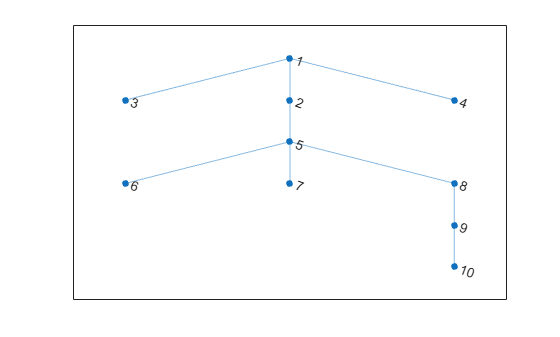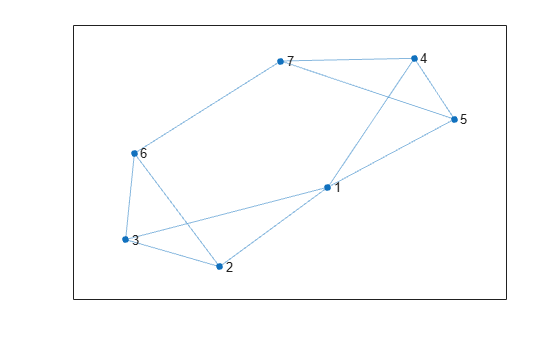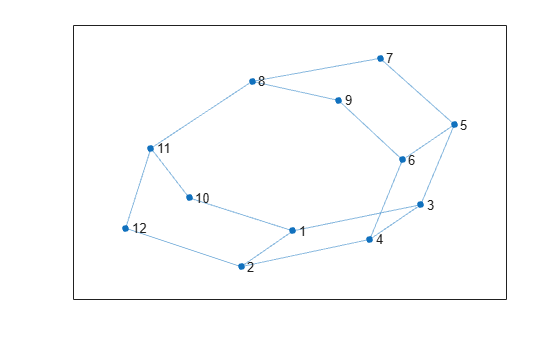# distances

Shortest path distances of all node pairs

## Syntax

``d = distances(G)``
``d = distances(G,s)``
``d = distances(G,s,t)``
``d = distances(___,'Method',algorithm)``

## Description

example

````d = distances(G)` returns a matrix, `d`, where `d(i,j)` is the length of the shortest path between node `i` and node `j`. If the graph is weighted (that is, `G.Edges` contains a variable `Weight`), then those weights are used as the distances along the edges in the graph. Otherwise, all edge distances are taken to be `1`.```

example

````d = distances(G,s)` restricts the source nodes to the nodes defined by `s`, such that `d(i,j)` is the distance from node `s(i)` to node `j`.```

example

````d = distances(G,s,t)` additionally restricts the target nodes to the nodes defined by `t`, such that `d(i,j)` is the distance from node `s(i)` to node `t(j)`.```

example

````d = distances(___,'Method',algorithm)` optionally specifies the algorithm to use in computing the shortest path using any of the input arguments in previous syntaxes. For example, if `G` is a weighted graph, then `distances(G,'Method','unweighted')` ignores the edge weights in `G` and instead treats all edge weights as `1`.```

## Examples

collapse all

Create and plot a graph.

```s = [1 1 1 2 5 5 5 8 9]; t = [2 3 4 5 6 7 8 9 10]; G = graph(s,t); plot(G)```Calculate the shortest path distance between all node pairs in the graph. Since the graph edges do not have weights, all edge distances are taken to be 1.

`d = distances(G)`
```d = 10×10 0 1 1 1 2 3 3 3 4 5 1 0 2 2 1 2 2 2 3 4 1 2 0 2 3 4 4 4 5 6 1 2 2 0 3 4 4 4 5 6 2 1 3 3 0 1 1 1 2 3 3 2 4 4 1 0 2 2 3 4 3 2 4 4 1 2 0 2 3 4 3 2 4 4 1 2 2 0 1 2 4 3 5 5 2 3 3 1 0 1 5 4 6 6 3 4 4 2 1 0 ```

`d` is symmetric because `G` is an undirected graph. In general `d(i,j)` is the length of the shortest path between node `i` and node `j`, and for undirected graphs this is equivalent to `d(j,i)`.

For example, find the length of the shortest path between node 1 and node 10.

`d(1,10)`
```ans = 5 ```

Create and plot a graph.

```s = [1 1 1 1 2 2 3 4 4 5 6]; t = [2 3 4 5 3 6 6 5 7 7 7]; G = graph(s,t); plot(G)```Find the shortest path distances from node 1, node 2, and node 3 to all other nodes in the graph.

`d = distances(G,[1 2 3])`
```d = 3×7 0 1 1 1 1 2 2 1 0 1 2 2 1 2 1 1 0 2 2 1 2 ```

Use `d` to find the shortest path distance from node 1 to node 7.

`d(1,7)`
```ans = 2 ```

Create and plot a graph.

```s = [1 1 1 2 2 3 3 4 5 5 6 7 8 8 10 11]; t = [2 3 10 4 12 5 4 6 6 7 9 8 9 11 11 12]; G = graph(s,t); plot(G)```Find the shortest path distances from nodes 5 and 7 to nodes 2 and 3.

```sources = [5 7]; targets = [2 3]; d = distances(G,sources,targets)```
```d = 2×2 3 1 4 2 ```

Use `d` to find the shortest path distance between node 7 and node 3. In this case, `d(i,j)` is the distance from node `sources(i)` to node `targets(j)`.

`d(2,2)`
```ans = 2 ```

Create and plot a directed graph with weighted edges.

```s = [1 1 1 2 5 3 6 4 7 8 8 8]; t = [2 3 4 5 3 6 4 7 2 6 7 5]; weights = [100 10 10 10 10 20 10 30 50 10 70 10]; G = digraph(s,t,weights); plot(G,'EdgeLabel',G.Edges.Weight)```Find the shortest path distance between all pairs of graph nodes.

`d = distances(G)`
```d = 8×8 0 90 10 10 100 30 40 Inf Inf 0 20 50 10 40 80 Inf Inf 110 0 30 120 20 60 Inf Inf 80 100 0 90 120 30 Inf Inf 120 10 40 0 30 70 Inf Inf 90 110 10 100 0 40 Inf Inf 50 70 100 60 90 0 Inf Inf 100 20 20 10 10 50 0 ```

Since `G` is a directed graph, `d` is not symmetric, and `d(i,j)` corresponds to the distance between nodes `i` and `j`. The `Inf` values in `d` correspond to nodes that are unreachable. For example, since node 1 has no predecessors, it is not possible to reach node 1 from any other node in the graph. So the first column of `d` contains many `Inf` values to reflect that node 1 is unreachable.

By default, `distances` uses the edge weights to compute the distances. Specify `'Method'` as `'unweighted'` to ignore the edge weights and treat all edge distances as 1.

`d1 = distances(G,'Method','unweighted')`
```d1 = 8×8 0 1 1 1 2 2 2 Inf Inf 0 2 4 1 3 5 Inf Inf 4 0 2 5 1 3 Inf Inf 2 4 0 3 5 1 Inf Inf 5 1 3 0 2 4 Inf Inf 3 5 1 4 0 2 Inf Inf 1 3 5 2 4 0 Inf Inf 2 2 2 1 1 1 0 ```

## Input Arguments

collapse all

Input graph, specified as either a `graph` or `digraph` object. Use `graph` to create an undirected graph or `digraph` to create a directed graph.

Example: `G = graph(1,2)`

Example: `G = digraph([1 2],[2 3])`

Source nodes, specified as one or more node indices or node names, or `'all'` to select all source nodes.

This table shows the different ways to refer to one or more nodes either by their numeric node indices or by their node names.

FormSingle NodeMultiple Nodes
Node index

Scalar

Example: `1`

Vector

Example: `[1 2 3]`

Node name

Character vector

Example: `'A'`

Cell array of character vectors

Example: `{'A' 'B' 'C'}`

String scalar

Example: `"A"`

String array

Example: `["A" "B" "C"]`

`s` and `t` must not specify nodes named `'all'` or `'Method'`, since these node names conflict with option names. Use `findnode` to instead pass in the node index for these cases.

Example: `distances(G,[1 2])`

Example: `distances(G,'all',[1 3 5])`

Target nodes, specified as one or more node indices or node names, or `'all'` to select all target nodes.

`s` and `t` must not specify nodes named `'all'` or `'Method'`, since these node names conflict with option names. Use `findnode` to instead pass in the node index for these cases.

Example: `distances(G,[1 2])`

Example: `distances(G,'all',[1 3 5])`

Shortest path algorithm, specified as one of the options in the table.

OptionDescription
`'auto'` (default)

The `'auto'` option automatically selects the algorithm:

• `'unweighted'` is used for `graph` and `digraph` inputs with no edge weights.

• `'positive'` is used for all `graph` inputs that have edge weights, and requires the weights to be nonnegative. This option is also used for `digraph` inputs with nonnegative edge weights.

• `'mixed'` is used for `digraph` inputs whose edge weights contain some negative values. The graph cannot have negative cycles.

`'unweighted'`

Breadth-First computation that treats all edge weights as `1`.

`'positive'`

Dijkstra algorithm that requires all edge weights to be nonnegative.

`'mixed'` (only for `digraph`)

Bellman-Ford algorithm for directed graphs that requires the graph to have no negative cycles.

While `'mixed'` is slower than `'positive'` for the same problem, `'mixed'` is more versatile as it allows some edge weights to be negative.

Note

For most graphs, `'unweighted'` is the fastest algorithm, followed by `'positive'`, and `'mixed'`.

Example: `distances(G,s,t,'Method','unweighted')`

## Output Arguments

collapse all

Shortest path distances between node pairs, returned as a matrix. The size of `d` is (# source nodes)-by-(# target nodes). A value of `Inf` indicates a path that does not exist.

## Tips

• The `shortestpath`, `shortestpathtree`, and `distances` functions do not support undirected graphs with negative edge weights, or more generally any graph containing a negative cycle, for these reasons:

• A negative cycle is a path that leads from a node back to itself, with the sum of the edge weights on the path being negative. If a negative cycle is on a path between two nodes, then no shortest path exists between the nodes, since a shorter path can always be found by traversing the negative cycle.

• A single negative edge weight in an undirected graph creates a negative cycle.